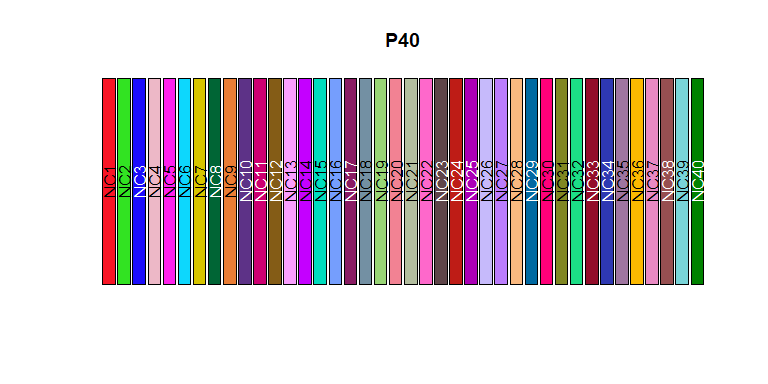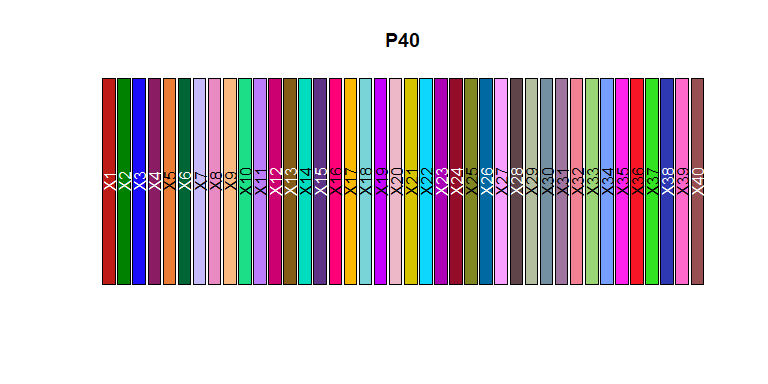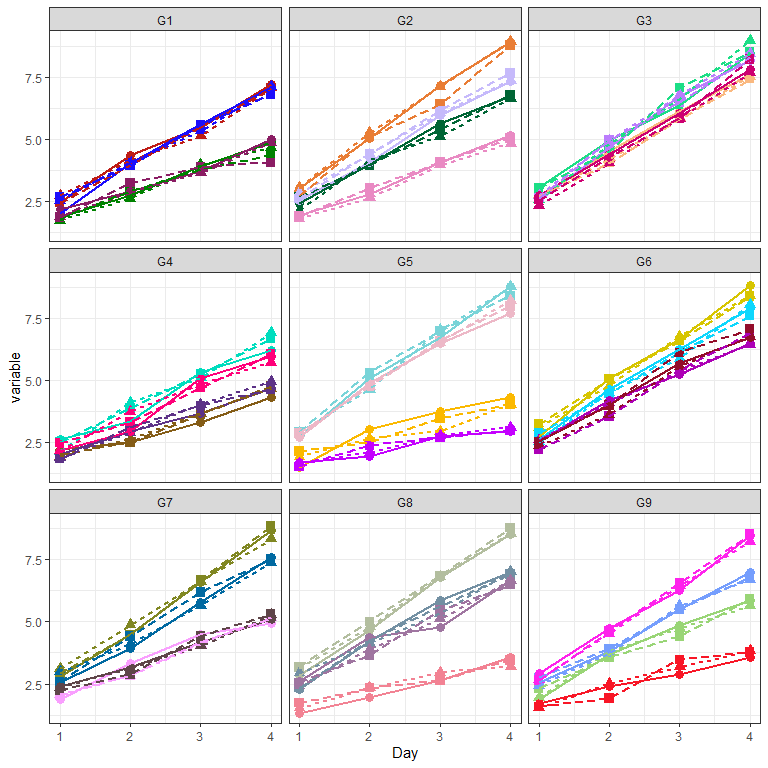Using Polychrome With ggplot

In this vignette, we describe how to use Polychrome palettes with the package ggplot2. The vignette will only run code if the ggplot2 package is available.

evalVignette <- requireNamespace("ggplot2", quietly = TRUE)
knitr::opts_chunk$set(eval = evalVignette) Getting Started We want to build a custom palette of 40 colors for this application, with each block of four consecutive colors being distinguishable. We start by constructing a new palette in the usual way. library(Polychrome) set.seed(935234) P40 <- createPalette(40, c("#FF0000", "#00FF00", "#0000FF"), range = c(30, 80)) swatch(P40)We achieve the goal of making the blocks of four colors being distinguishable by first sorting by hue, and then rearranging them into four-blocks. P40 <- sortByHue(P40) P40 <- as.vector(t(matrix(P40, ncol=4))) swatch(P40)Here is the key point of this entire vignette: By default, Polychrome gives names to each of the colors in a palette. But, in ggplot, named colors will only be applied if they match the levels of an appropriate factor in the data. The simplest solution is to remove the names: names(P40) <- NULL Simulating Complex Data For illustration purposes, we simulate a data set with a moderately complex structure. Specifically, we assume that we have • Nine groups of samples, • Four samples in each group, • Three replicate experiments for each sample, and • Measurements repeated over four days. Here is the simulated design of the data set. ## Nine groups NG <- 9 gp <- paste("G", 1:NG, sep = "") length(gp) ##  9 ## Four Subjects per group ## 36 Subjects = 9 groups * 4 subjects/group sid <- paste(rep(LETTERS[1:2], each=26), c(LETTERS, LETTERS), sep="")[1:(4*NG)] length(sid) ##  36 ## Three Reps per subject ## 108 Experiments reps = factor(rep(c("R1", "R2", "R3"), times = length(sid))) length(reps) ##  108 ## Each experiment with measurements on four Days, so 432 data rows daft <- data.frame(Day = rep(1:4, each=length(reps)), Group = factor(rep(rep(gp, each=12), times = 4)), Subject = factor(rep(rep(sid, each = 3), times=4)), Rep = factor(rep(reps, times = 4))) dim(daft) ##  432 4 summary(daft) ## Day Group Subject Rep ## Min. :1.00 G1 : 48 AA : 12 R1:144 ## 1st Qu.:1.75 G2 : 48 AB : 12 R2:144 ## Median :2.50 G3 : 48 AC : 12 R3:144 ## Mean :2.50 G4 : 48 AD : 12 ## 3rd Qu.:3.25 G5 : 48 AE : 12 ## Max. :4.00 G6 : 48 AF : 12 ## (Other):144 (Other):360 Now we add simulated “measurements” taken on each replicate of each subject on each of four days. ## Linear model with noise, ignoring group beta <- runif(length(sid), 0.5, 2) ## "Measured" variable attach(daft) daft$variable <- rnorm(nrow(daft), 0, 0.2) + 1  + beta[as.numeric(Subject)]*Day
detach()

Plotting the results.

library(ggplot2)
ggplot(daft, aes(x = Day, y = variable, colour = as.factor(Subject))) +
geom_point(aes(shape = as.factor(Rep)), size = 3) +
geom_line(aes(linetype = as.factor(Rep)), size = 0.8) +
facet_wrap(. ~ Group, ncol = 3)+
theme_bw() + theme(legend.position="none")+
scale_color_manual(values = P40)A faceted plot, colored by subject.